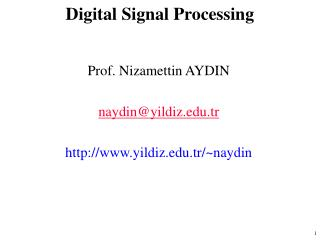DownloadDownload PresentationDigital Signal Processing

# Digital Signal Processing

Download Presentation## Digital Signal Processing

- - - - - - - - - - - - - - - - - - - - - - - - - - - E N D - - - - - - - - - - - - - - - - - - - - - - - - - - -
##### Presentation Transcript

1. Digital Signal Processing Prof. Nizamettin AYDIN naydin@yildiz.edu.tr http://www.yildiz.edu.tr/~naydin

2. Digital Signal Processing Lecture 13 Digital Filtering of Analog Signals

3. READING ASSIGNMENTS • This Lecture: • Chapter 6, Sections 6-6, 6-7 & 6-8 • Other Reading: • Recitation: Chapter 6 • FREQUENCY RESPONSE EXAMPLES • Next Lecture: Chapter 7

4. LECTURE OBJECTIVES x(t) A-to-D x[n] y[n] D-to-A y(t) FIR • Two Domains: Time & Frequency • Track the spectrum of x[n] thru an FIR Filter: Sinusoid-IN gives Sinusoid-OUT • UNIFICATION: How does Frequency Response affect x(t) to produce y(t) ?

5. TIME & FREQUENCY FIR DIFFERENCE EQUATION is the TIME-DOMAIN

6. Ex: DELAY by 2 SYSTEM x[n] y[n] x[n] y[n]

7. DELAY by 2 SYSTEM x[n] y[n] k = 2 ONLY x[n] y[n]

8. GENERAL DELAY PROPERTY ONLY ONE non-ZERO TERM for k at k = nd

9. FREQ DOMAIN --> TIME ?? x[n] y[n] x[n] y[n] • START with

10. FREQ DOMAIN --> TIME EULER’s Formula

11. PREVIOUS LECTURE REVIEW MAG PHASE • SINUSOIDAL INPUT SIGNAL • OUTPUT has SAME FREQUENCY • DIFFERENT Amplitude and Phase • FREQUENCY RESPONSE of FIR • MAGNITUDE vs. Frequency • PHASE vs. Freq • PLOTTING

12. FREQ. RESPONSE PLOTS • DENSE GRID (ww) from -p to +p • ww = -pi:(pi/100):pi; • HH = freqz(bb,1,ww) • VECTOR bb contains Filter Coefficients • DSP-First: HH = freekz(bb,1,ww)

13. PLOT of FREQ RESPONSE RESPONSE at p/3

14. EXAMPLE 6.2 x[n] y[n]

16. EXAMPLE: COSINE INPUT x[n] y[n]

17. EX: COSINE INPUT (ans-1)

18. EX: COSINE INPUT (ans-2)

19. SINUSOID thru FIR • IF • Multiply the Magnitudes • Add the Phases

20. LTI Demo with Sinusoids y[n] x[n] FILTER

21. DIGITAL “FILTERING” x(t) A-to-D x[n] y[n] D-to-A y(t) • SPECTRUM of x(t) (SUM of SINUSOIDS) • SPECTRUM of x[n] • Is ALIASING a PROBLEM ? • SPECTRUM y[n](FIR Gain or Nulls) • Then, OUTPUT y(t) = SUM of SINUSOIDS

22. FREQUENCY SCALING x(t) A-to-D x[n] y[n] D-to-A y(t) • TIME SAMPLING: • IF NO ALIASING: • FREQUENCY SCALING

23. 11-pt AVERAGER Example x(t) A-to-D x[n] y[n] D-to-A y(t) ? 250 Hz 25 Hz

24. D-A FREQUENCY SCALING x(t) A-to-D x[n] y[n] D-to-A y(t) • RECONSTRUCT up to 0.5fs • FREQUENCY SCALING • TIME SAMPLING:

25. TRACK the FREQUENCIES x(t) A-to-D x[n] y[n] D-to-A y(t) • 250 Hz • 25 Hz • 0.5p • .05p • 0.5p • .05p • 250 Hz • 25 Hz Fs = 1000 Hz NO new freqs

26. 11-pt AVERAGER NULLS or ZEROS

27. EVALUATE Freq. Response

28. EVALUATE Freq. Response MAG SCALE PHASE CHANGE fs = 1000

29. DIGITAL FILTER EFFECTIVE RESPONSE LOW-PASS FILTER

30. FILTER TYPES • LOW-PASS FILTER (LPF) • BLURRING • ATTENUATES HIGH FREQUENCIES • HIGH-PASS FILTER (HPF) • SHARPENING for IMAGES • BOOSTS THE HIGHS • REMOVES DC • BAND-PASS FILTER (BPF)

31. B & W IMAGE

32. B&W IMAGE with COSINE FILTERED: 11-pt AVG

33. FILTERED B&W IMAGE LPF: BLUR

34. ROW of B&W IMAGE BLACK = 255 WHITE = 0

35. FILTERED ROW of IMAGE ADJUSTED DELAY by 5 samples